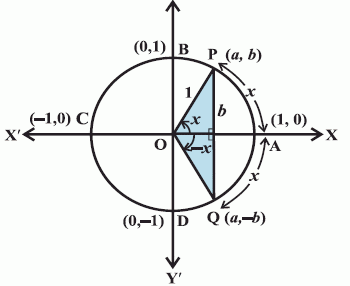Name: ___________________Date:___________________

 Email us to get an instant 20% discount on highly effective K-12 Math & English kwizNET Programs!

### High School Mathematics - 210.8 Signs of Trigonometric Functions for Negative Angles

 θ sin θ cos θ tan θ cosec θ sec θ cot θ 0° 0 1 0 n.d. 1 n.d. 30° 1/2 Ö3/2 1/Ö3 2 2/Ö3 Ö3 45° 1/Ö2 1/Ö2 1 Ö2 Ö2 1 60° Ö3/2 1/2 Ö3 2/Ö3 2 1/Ö3 90° 1 0 n.d. 1 n.d. 0
Signs of trigonometric functions for negative angles
Let P(x,y) be a point on the unit circle with centre at origin such that angle AOP = θ. If angle AOQ = -θ, then the co-ordinates of the point Q will be (x,-y). Hence
cos(-θ) = x = cosθ
sin(-θ) = -y = -sinθI II III IV sinθ + + - - cosθ + - - + tanθ + - + - cosecθ + + - - secθ + - - + cotθ + - + -
In the first quadrant, as θ increases from 0 to π/2, sinθ increases from 0 to 1.
In the second quadrant, as θ increases from to π/2 to π,to π sinθ decreases from 1 to 0.
In the third quadrant, as θ increases from π to 3π/2, sinθ decreases from 0 to -1.
In the fourth quadrant, sinθ increases from -1 to 0, as θ increases from 3π/2 to 2π.

Directions: Solve the following.
 Q 1: State the quadrants in which cos x = .7331 may lie.II, IIII, III, IV Question 2: This question is available to subscribers only!